### Site Information
Loading... Please wait...
• Compare
• My Account
• All prices are in USD

# How much Spray Foam do I need? Determining Area

Posted by on

#### Answer: We haven’t a clue.

Why can’t we answer this? Because the square footage of the living area (usually the area of the floor) doesn’t tell us the area of the walls, floors and ceiling that need to be covered. So we need to do some calculations to figure out the area to be covered.

### First some definitions

• Length – This is measuring in a straight line (1 dimension). Linear Feet is a distance of a straight line measured in feet.
Units: Feet (Linear Feet), or Inches
• Area – This is measurement of a shape that has both length and width (2 dimensions), like a square or rectangle. For a simple shape you multiply the lengths of the two sides.
Units: Square Feet or Square Inches
• Volume – This is measurement of a shape that has length, width, and height (3 dimensions). For a simple box, you multiply all 3 lengths together.
Units: Cubic Feet or Cubic Inches

When working with any of these make sure that you use the same units for all your measurements. If you have a mix of feet and inches convert everything to feet or everything to inches.

### So where to start.

Look at the building you want to insulate. Determine exactly which walls, floor or ceilings that you want to apply foam to. We’re going to calculate the area of each surface and add them together to see how big an area we want to cover.

#### Take a look at our example building.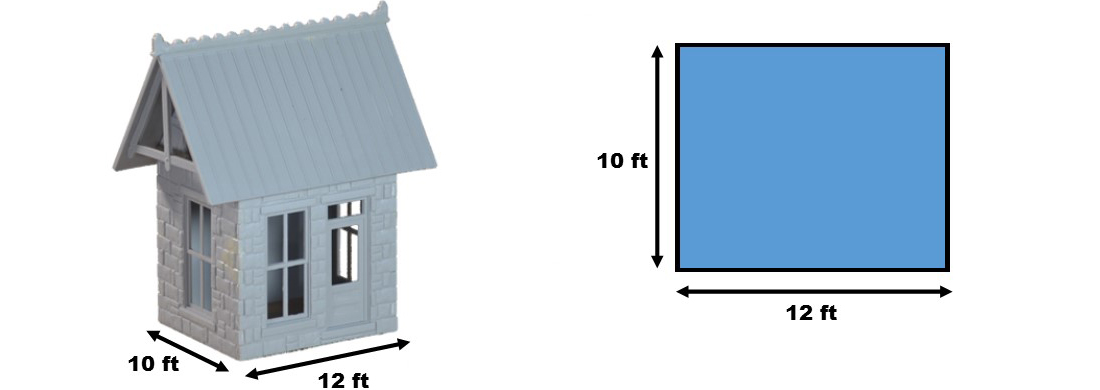It has 4 walls, one side is 12 ft long and the other side is 10 ft long.

So the total area of the floor is 10 ft x 12 ft which gives us 120 sq ft (square feet) of floor area.

That's great if we want to insulate the floor, but we want to insulate the walls.

### Figuring out the area of the walls.

Instead of using length times width to calculate the floor area we need to look at each section of wall and calculate the area of each wall section separately.

Imagine that we can separate the walls and lay them flat. We can then figure out the area of each section and add them together for a grand total.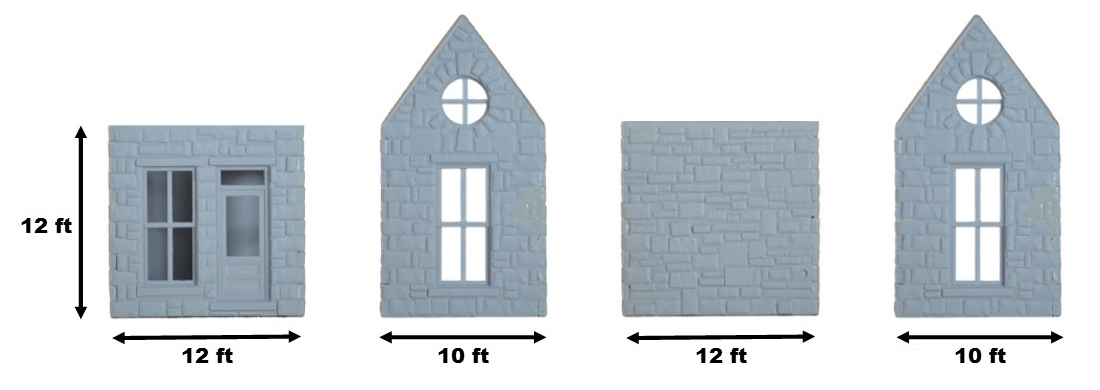The square sections are easy, just multiply length times width (or in this case length times height) to get the area of that section.

But wait, not all wall sections are square. In that case it's easier to split up the wall into several sections and calculate each area separately.

For a wall with a roof peak, we split it into two sections, a rectangle and a triangle.

• The rectangle is length times height, easy.
• The triangle, a little bit harder. Going back to that Junior High geometry class, remember that the area of a triangle is 1/2 of length times height. Not so hard, is it?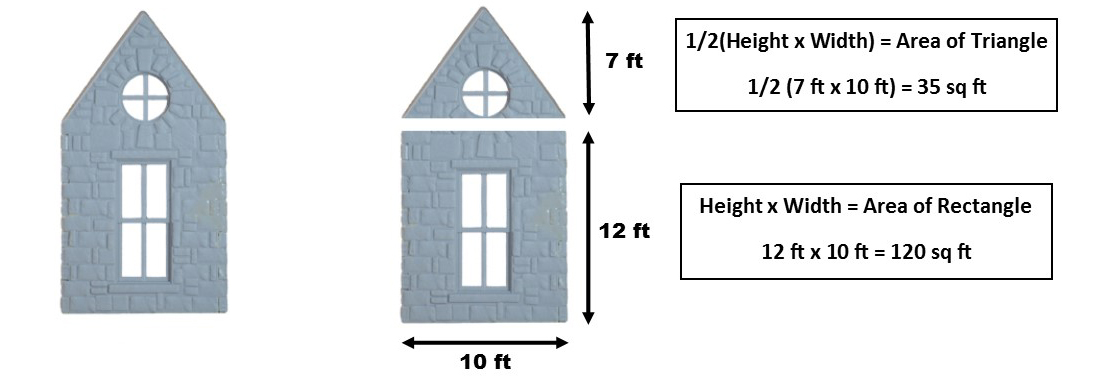#### So now we figured out how to do this, we just need to do the calculations.

 Wall Section Dimensions Formula Area Front 12 ft x 12 ft length x height 144 sq.ft. Side Base 10 ft x 12 ft length x height 120 sq.ft. Peak (Triangle) 10 ft x 7 ft 1/2(length x height) 35 sq.ft. Back 12 ft x 12 ft length x height 144 sq.ft. Side Base 10 ft x 12 ft length x height 120 sq.ft. Peak (Triangle) 10 ft x 7 ft 1/2(length x height) 35 sq.ft Total Area = 598 sq.ft.

Our total wall area is 598 square feet. This includes windows and doors.

Now let's refine this a little. You don't want to insulate the windows and doors, do you? To take away for the spaces you can't insulate, figure the area of all the windows and doors (just like we just did for the walls) and subtract it from the above total. I did this and figured out our windows cover 94 sq ft of area.

Wall Area - Window Area = Area to be Insulated

598 sq.ft. - 94 sq.ft. = 504 sq.ft.

Our total area we want to insulate is 504 sq.ft.

### How Much Foam Do I Need?

If you look at a spray foam kit such as a Dow Froth Pak, you'll see that it's rated to cover so many square feet at 1 inch thick. This is what is known as a "Board Foot" or"bd ft".

Remember how we said not to mix feet and inches when making these calculations. With board feet we ignore this.

As this picture shows it takes 12 board feet to be the same as 1 cubic foot.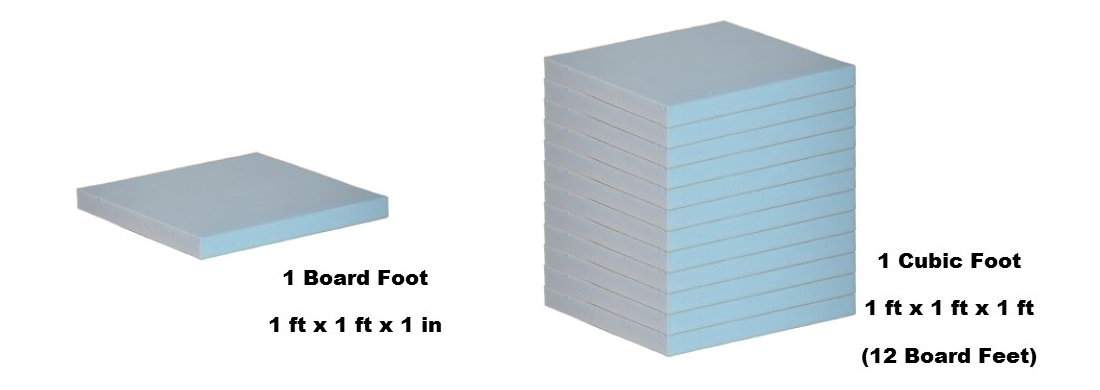To determine how much foam you'll need, you need to know 2 things, the area to be insulated (square feet) and how thick you want your insulation (inches).

Multiply both of these together and you get the number of board feet of insulation that you need to buy.

To insulate 1 inch thick

504 sq.ft. x 1 in = 504 Board Feet

To insulate 2 inch thick

504 sq.ft. x 2 in = 1008 Board Feet

For 1 inch of foam, 1 Dow Froth Pak 650 (650 bd ft) will cover our building with a little left over.

For 2 inches of foam, 1 Dow Froth Pak 650 and 2 Dow Froth Pak 210's (650+210+210=1070 bd ft) should work.